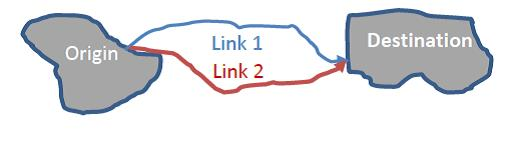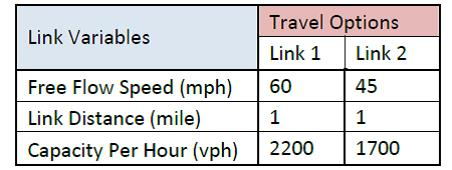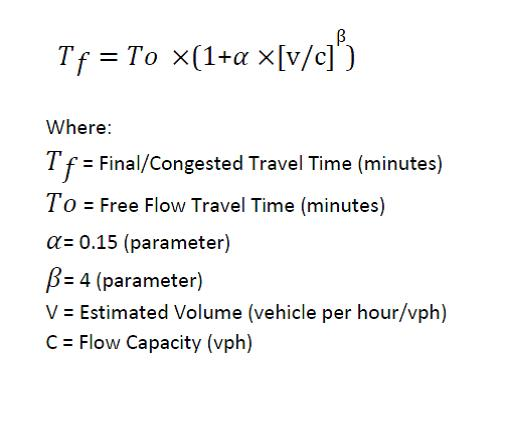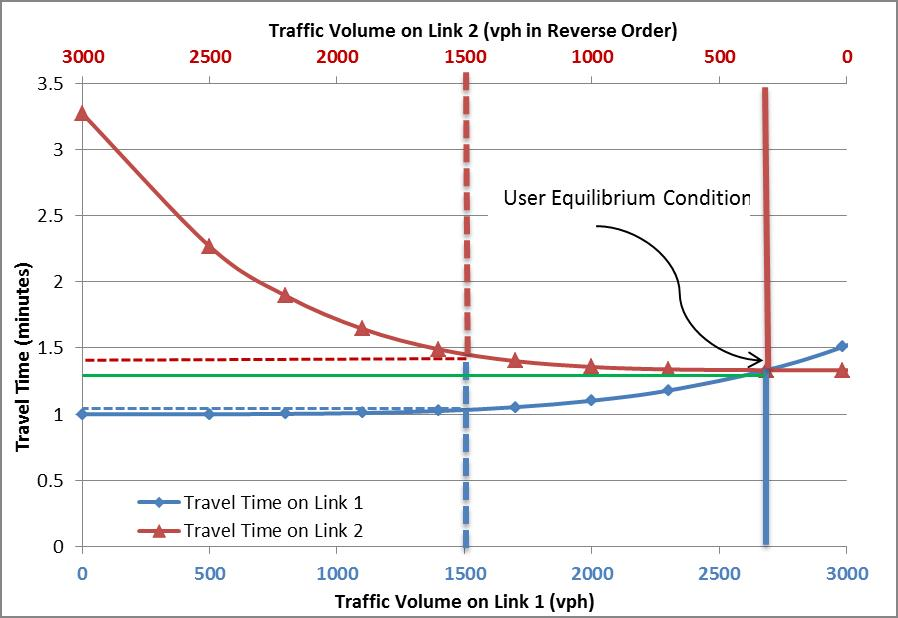# User Equilibrium

## # Summary

Although the term user equilibrium (UE) is not mentioned in Wardrop’s paper^1^, in the context of transportation modeling, the term is used to describe a route choice assumption formally proposed by Wardrop: “The journey times on all the routes actually used are equal and less than those which would be experienced by a single vehicle on any unused routes”. This criterion is also known as Wardrop’s first principle.

This condition of UE can be described with an example case where an origin destination (OD) pair is connected by two one-way links representing two different routes. Illustrated in Figure 1, the assumed traffic demand between these OD is 3000 vehicle per hour (vph). Fixed characteristics of links utilized in the calculation are listed in Table 1. Travel times on these links are estimated by using a typical Bureau of Public Roads volume delay function shown in equation below where travel time is an increasing function of flow. This type of flow dependent functions for estimating travel time are also called link cost function.

Figure 1: Origin Destination Travel OptionsFigure 2: An Example of User Equilibrium Condition